# Tracking

For horizontal north-south axis tracker, this procedure computes the ideal tracker angle φτ, taking into consideration backtracking shade avoidance strategy and the tracker’s mechanical limits of travel. This procedure is generalized for a tracker whose N-S axis is skewed by a tracker yaw angle γτ (azimuth angle).

The coordinate system used in this suite of algorithms is the “North-East-Down” convention (NED), as follows:

• Solar azimuth angle is measured from North (N=0°, E=90°, S=180°, W=270°)

The other angles are positive when rotating counter-clockwise looking towards the origin.

• Tracker roll (tilt) angle is positive East, negative West, 0° is horizontal (facing up)
• Tracker yaw angles are positive east (e.g. 0° is N-S; 10° has a slight NNW-SSE orientation)

This is illustrated in Figure 5.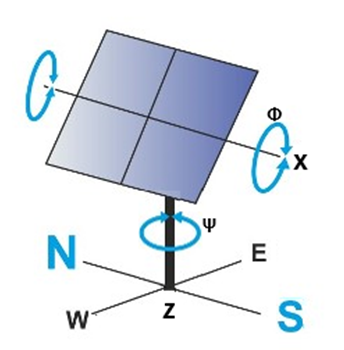### Figure 5. Modified Tracker Coordinate System

This procedure returns the tracker angle, as well as the effective tilt and azimuth angles, rotated in the reference
frame of a basic fixed-tilt array. In the case of a horizontal north-south tracker with a yaw angle of 10° degrees
east with the tracker set at an evening angle 45° to the east, the effective tilt β and azimuth γ would
be +45° be and +80°, respectively. The derivation of the rotation angle for optimum tracking of a single-axis

PlantPredict only supports a horizontal tracker (where the pitch angle is zero). This section summarizes the referenced equations into a form easily implanted in source code.

Note: Unlike in the NREL technical report, the incidence angle is calculated separately in order to have a generalized solution for both fixed-tilt and tracking array configurations.

## Inputs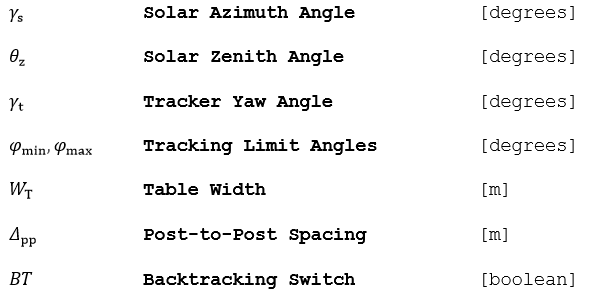## Outputs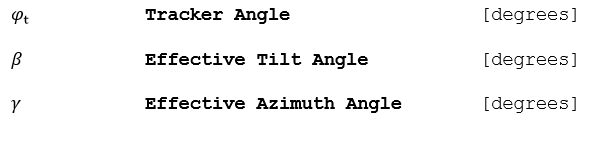## Algorithm (True Tracking)

1.) Convert the solar azimuth and yaw angles into the NED reference frame.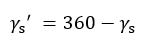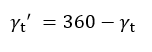2.) Find the ideal tracker angle, with no backtracking or mechanical stops.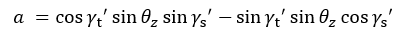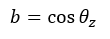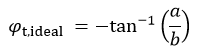3.) If the solar azimuth angle is not between (-90°, +90°), switch the sign of the ideal tracker angle: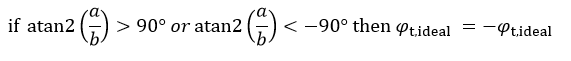## Algorithm (Backracking)

If backtracking is activated (as it would typically be for PV technologies using bypass diodes), then compute
the modified tracker angle with shade avoidance:

1.) Find the cutoff tracking angle where the row-to-row shading begins, which depends on the ground coverage
ratio (tracker width / post-to-post spacing)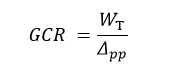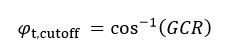2.) If the cutoff angle is not reached, then tracking is unaffected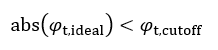3.) Else, invoke the backtracking algorithm, taking care of the sign of the tracker angle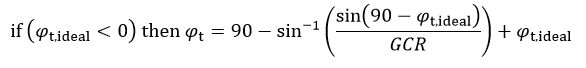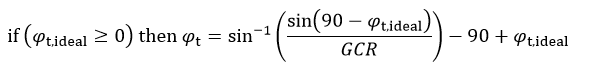## Algorithm (Tracker Limits)

1.) If the tracker limits are reached, the tracker stops moving.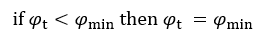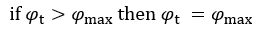2.) Report the effective tilt and azimuth angles. An east-west tracker is defined to have an azimuth of 0°
if the tracker axis is north-south (azimuth is defined relative to the tracking axis, not the collector);
hence in the morning, the tracker has an effective collector azimuth of 90° (facing east), and in the afternoon,
the effective collector azimuth is 270° (facing west).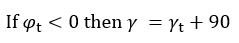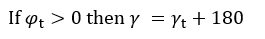3.) Report the effective tilt angle as the absolute value of the tracker angle (simplification only valid for
horizontal trackers).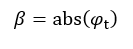## Reference

Marion, William F. and Dobos, Aron P. “Rotation Angle for the Optimum Tracking of One-Axis Trackers.”NREL. July 2013. NREL/TP-6A20-58891, 2003